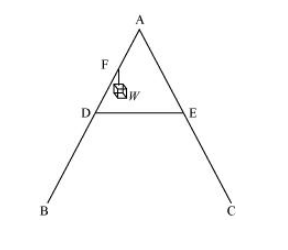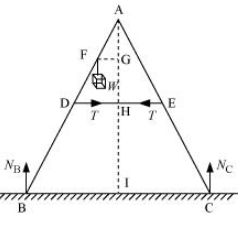# As shown in Fig.7.40, the two sides of a step ladder BA and CA are 1.6 m long and hinged at A.

Question:

As shown in Fig.7.40, the two sides of a step ladder BA and CA are $1.6 \mathrm{~m}$ long and hinged at A A rope DE, $0.5 \mathrm{~m}$ is tied half way up. A weight $40 \mathrm{~kg}$ is suspended from a point $F, 1.2 \mathrm{~m}$ from $B$ along the ladder BA. Assuming the floor to be frictionless and neglecting the weight of the ladder, find the tension in the rope and forces exerted by the floor on the ladder. (Take $g=9.8 \mathrm{~m} / \mathrm{s}^{2}$ )

(Hint: Consider the equilibrium of each side of the ladder separately.)Solution:

The given situation can be shown as:NB = Force exerted on the ladder by the floor point B

NC = Force exerted on the ladder by the floor point C

= Tension in the rope

BA = CA = 1.6 m

DE = 0. 5 m

BF = 1.2 m

Mass of the weight, m = 40 kg

Draw a perpendicular from A on the floor BC. This intersects DE at mid-point H.

$\triangle \mathrm{ABI}$ and $\triangle \mathrm{AlC}$ are similar

$\therefore \mathrm{BI}=\mathrm{IC}$

Hence, $I$ is the mid-point of $B C$.

$D E \| B C$

$B C=2 \times D E=1 \mathrm{~m}$

$\mathrm{AF}=\mathrm{BA}-\mathrm{BF}=0.4 \mathrm{~m} \ldots(i)$

$D$ is the mid-point of $A B$.

Hence, we can write:

$\mathrm{AD}=\frac{1}{2} \times \mathrm{BA}=0.8 \mathrm{~m}$  $\ldots(i i)$

Using equations (i) and (ii), we get:

FE = 0.4 m

Hence, F is the mid-point of AD.

$\mathrm{FG} \| \mathrm{DH}$ and $\mathrm{F}$ is the mid-point of $\mathrm{AD}$. Hence, $\mathrm{G}$ will also be the mid-point of $\mathrm{AH}$.

$\triangle \mathrm{AFG}$ and $\triangle \mathrm{ADH}$ are similar

$\therefore \frac{\mathrm{FG}}{\mathrm{DH}}=\frac{\mathrm{AF}}{\mathrm{AD}}$

$\frac{\mathrm{FG}}{\mathrm{DH}}=\frac{0.4}{0.8}=\frac{1}{2}$

$\mathrm{FG}=\frac{1}{2} \mathrm{DH}$

$=\frac{1}{2} \times 0.25=0.125 \mathrm{~m}$

In $\triangle A D H$ :

$\mathrm{AH}=\sqrt{\mathrm{AD}^{2}-\mathrm{DH}^{2}}$

$=\sqrt{(0.8)^{2}-(0.25)^{2}}=0.76 \mathrm{~m}$

For translational equilibrium of the ladder, the upward force should be equal to the downward force.

$N_{\mathrm{C}}+N_{\mathrm{B}}=m g=392 \ldots$ (iii)

For rotational equilibrium of the ladder, the net moment about $A$ is:

$-N_{\mathrm{B}} \times \mathrm{BI}+m \mathrm{~g} \times \mathrm{FG}+N_{\mathrm{C}} \times \mathrm{Cl}+T \times \mathrm{AG}-T \times \mathrm{AG}=0$

$-N_{\mathrm{B}} \times 0.5+40 \times 9.8 \times 0.125+N_{\mathrm{C}} \times(0.5)=0$

$\left(N_{\mathrm{C}}-N_{\mathrm{B}}\right) \times 0.5=49$

$N_{\mathrm{C}}-N_{\mathrm{B}}=98$ $\ldots(i v)$

Adding equations (iii) and (iv), we get:

$N_{\mathrm{C}}=245 \mathrm{~N}$

$N_{\mathrm{B}}=147 \mathrm{~N}$

For rotational equilibrium of the side $A B$, consider the moment about $A$.

$-N_{\mathrm{B}} \times \mathrm{BI}+m \mathrm{~g} \times \mathrm{FG}+T \times \mathrm{AG}=0$

$-245 \times 0.5+40+9.8 \times 0.125+T \times 0.76=0$

$0.76 T=122.5-49$

$\therefore T=96.7 \mathrm{~N}$